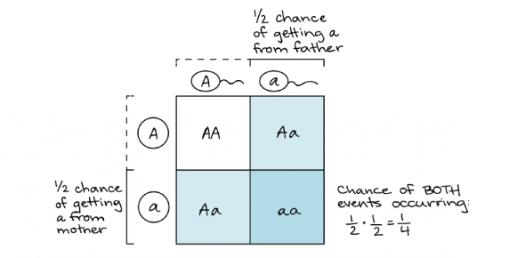Biology: Probability Of Inheritance Quiz!

8 Questions | Total Attempts: 498SettingsWhat do you know about biology? An example of biology using probability is the Punnet square. It is a square diagram that is used to predict the genotypes of a particular cross or breeding experiment. The diagram is used by biologists to determine the probability of offspring possessing a specific genotype. These tablets can be used to examine genotypical outcomes. Take this quiz and see how much you know about biology and probability.

• 1.
Punnett squares are used by geneticists to determine the probability of different offspring genotypes. What letter(s) belong in the lower right box if one parent is homologous recessive and the other it heterozygous?
• A.

AA

• B.

Aa

• C.

Aa

• D.

A-

• 2.
In a certain species of pine trees, short needles (S) are dominant to long needles (s). According to the Punnett square, what is the probability of an offspring having long needles?
• A.

25%

• B.

50%

• C.

75%

• D.

100%

• 3.
A heterozygous tall (Tt) plant is crossed at with a short plant (tt). The probability that offspring plants will be tall is...
• A.

25%

• B.

50%

• C.

75%

• D.

100%

• 4.
One cat carries heterozygous, long-haired traits (SS), and its mate carries homozygous short-haired traits (ss). Use a Punnett square to determine the probability of one of their offspring having long hair.
• A.

25%

• B.

50%

• C.

75%

• D.

100%

• 5.
An example of a heterozygous genotype is...
• A.

Tt

• B.

Tt

• C.

TT

• D.

Aa

• 6.
Tracey has three brothers. One of Tracey's brothers has sickle-cell anemia, a disease caused by a recessive allele. She, her parents, and the other children do not have it. If Tracey's parents have another child, what is the chance that the child will have sickle-cell anemia?
• A.

0%

• B.

25%

• C.

50%

• D.

75%

• 7.
In pepper plants, the allele for hot flavor (H) is dominant to the allele for mild flavor(h).  A farmer crosses a homozygous dominant plant with a recessive (mild) plant.What percentage of the offspring from this cross will have hot flavor?
• A.

25%

• B.

50%

• C.

75%

• D.

100%Back to top